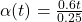Question

A disk of radius 25.0cm turns about an axis through the center. The pull on the string produces a linear acceleration a(t)=At on the ball The disk starts from rest and after 3 seconds, linear a(3)=1.80m/s2. Find A and then write an expression for the angular acceleration α(t).

1.The value for  A  is A= 0.6

The angular acceleration is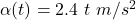Explanation:

From the question we are told that

The radius of the disk is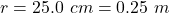The linear acceleration is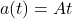At time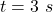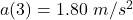Generally angular acceleration is  mathematically represented as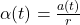Now  at t = 3 seconds

a(3) =  A *  3

=>      1.80 =  A  *  3

=.>       A =  0.6

So  therefore

a(t) =  0.6 t

Now  substituting this into formula for angular acceleration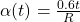substituting for  r